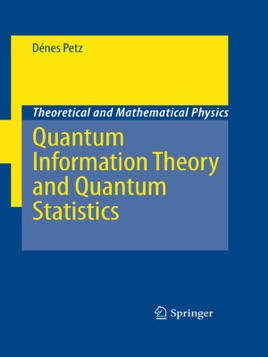• USD 69.99

## Descripción de editorial

Based on lectures given by the author, this book focuses on providing reliable introductory explanations of key concepts of quantum information theory and quantum statistics - rather than on results. The mathematically rigorous presentation is supported by numerous examples and exercises and by an appendix summarizing the relevant aspects of linear analysis. Assuming that the reader is familiar with the content of standard undergraduate courses in quantum mechanics, probability theory, linear algebra and functional analysis, the book addresses graduate students of mathematics and physics as well as theoretical and mathematical physicists. Conceived as a primer to bridge the gap between statistical physics and quantum information, a field to which the author has contributed significantly himself, it emphasizes concepts and thorough discussions of the fundamental notions to prepare the reader for deeper studies, not least through the selection of well chosen exercises.